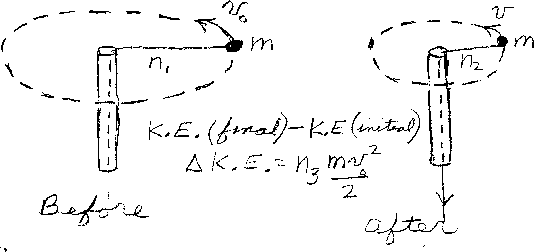Problem D9: A small ball of mass m is initially moving in a circle with a constant speed. The radius of the circle is n1, and its speed is v0 as shown in the figure. The string holding the ball passes through a pipe. Now the string is pulled through the pipe which shortens the radius of the motion. The string is pulled until the radius is n2. The motion is still circular, but with a faster speed. How much has the kinetic energy of the ball increased due to pulling the string? If the increase in kinetic energy is K.E.(final)-K.E.(initial) = Δ K.E. = n3 mv02/2, what is n3. Note that n1 and n2 have the same units of distance, and n3 is unitless.n1 = n2 = Input n3:
If you are currently in my class, you can record your grade by entering your name and student ID number (without the leading zeros) below and clicking on "record grade".
 First Name = Last Name = ID = Problem: